Next: Zhukovskii's Hypothesis Up: Incompressible Aerodynamics Previous: Theorem of Kutta and

# Cylindrical Airfoils

For the moment, let us work in the complex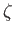-plane, where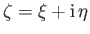. Consider a cylindrical airfoil with a circular cross-section of radius, centered on the origin, that is situated in a uniform, high Reynolds number wind of speedwhose direction subtends a (clockwise) angle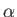with the negative-axis. Letbe the circulation of air around the airfoil. A slight generalization of the analysis of Section 6.4 reveals that the appropriate complex velocity potential is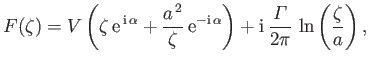(9.14)

whereas the associated stream function takes the form(9.15)

where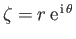. It follows that(9.16)

Comparison with Equation (9.6) (with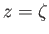) reveals that(9.17)

Hence, Equations (9.10) and (9.13) yield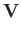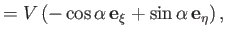(9.18)(9.19)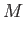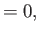(9.20)

whereis the wind vector,the lift vector, and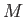the moment of the lift vector about the origin. We conclude that, for a cylindrical airfoil of circular cross-section, the lift vector is normal to the wind vector, and the line of action of the lift passes through the centroid of the cross-section (because the lift generates zero moment about the origin). (See Figure 9.1.)Of course, a cylindrical airfoil of circular cross-section is completely unrealistic, because its back side (i.e., the side opposite to that from which the wind is incident) is not sufficiently streamlined to prevent boundary layer separation. (See Chapter 8.) However, as described in Section 6.7, we can use the conformal transformation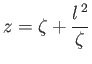(9.21)

to transform a cylinder of circular cross-section in the-plane to a cylinder of elliptical cross-section in the-plane. (Note that both cross-sections have centroids located at the origin.) Moreover, a cylindrical airfoil of elliptical cross-section that is sufficiently elongated, and whose major axis subtends a sufficiently small angle with the incident wind direction, constitutes a realistic airfoil, because its back side is, for the most part, closely aligned with the external flow.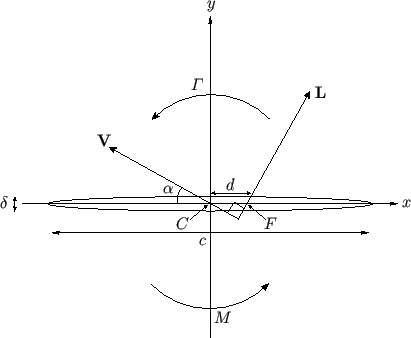An elliptical airfoil of width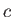and thickness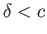, as shown in Figure 9.2, is obtained when the parametersand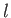are given the following values:(9.22)(9.23)

In this case, the surface of the airfoil satisfies the parametric equations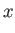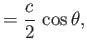(9.24)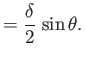(9.25)

In particular, the airfoil's leading and trailing edges correspond to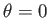and, respectively.

According to Equations (9.16) and (9.21), the complex velocity in the-plane is given by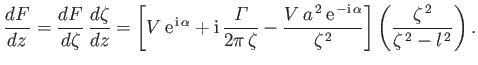(9.26)

Thus, on the airfoil surface, where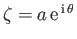, we obtain(9.27)

A long way from the airfoil,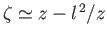, so that Equation (9.26) reduces to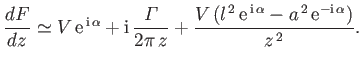(9.28)

A comparison with Equation (9.6) reveals that the circulation of air around the airfoil takes the same value,, in both the complex- and-planes. In other words, the conformal transformation (9.21) does not modify the circulation. The transformation also does not modify the external wind speed or direction [because, from (9.16) and (9.28),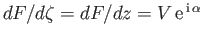at very largeand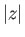]. On the other hand, it is clear that the constant, which takes the value zero in the complex-plane, takes the value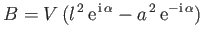(9.29)

in the complex-plane. Hence, Equations (9.10) and (9.13) reveal that(9.30)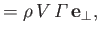(9.31)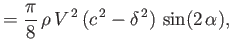(9.32)

whereis the wind vector,the lift vector, andthe moment of the lift vector about the origin. Here,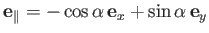(9.33)

is a unit vector parallel to the incident wind direction, and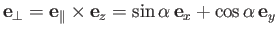(9.34)

is a unit vector perpendicular to the wind direction. We conclude that, for a cylindrical airfoil of elliptic cross-section, the lift vector is normal to the wind vector, but the line of action of the lift intersects the major axis of the airfoil a distance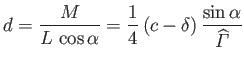(9.35)

in front of the cross-section's centroid,, where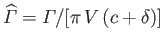. (See Figure 9.2.) Incidentally, the point,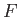, at which the line of action of the lift intersects the airfoil's major axis is conventionally termed the focus of the airfoil.Next: Zhukovskii's Hypothesis Up: Incompressible Aerodynamics Previous: Theorem of Kutta and
Richard Fitzpatrick 2016-03-31